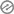# PolygonsSubject : Mathematics

Class : Basic 6Topic : Polygons

Content :

Polygons are shapes.

Shapes are forms or appearance.Appearance are looks like the appearance of your bother or the look of your door or the shape of your bag

Shapes have two major groups which are 2 dimensional shapes (2D) and three dimensional shapes6(3D)

2D shapes are plain flat shapes.

Examples of 2 dimensional polygons

1. Triangles : These are polygons with three sides and three interior angles eg scalane triangle, isosceles triangle, equilateral triangle etc

2. Quadrilateral : These are polygons with four sides and four interior angles eg kite, square,
rectangle, parallelogram

3. Pentagon : This is a polygon with five sides and angles

4. Hexagon : This is a polygon with six interior angles and six sides

5. Heptagon : this is a polygon with seven sides and seven interior angles

6. Octagon : A polygon with eight sides and angles

7. Nonagon : This is a polygon with nine sides and nine angles

8. Decagon : This is a polygon with ten sides and ten angles.Classwork

Draw the following polygons

1. Triangle

2. Nonagon3. Pentagon

4. Decagon

Sharing is Caring – Pass It On